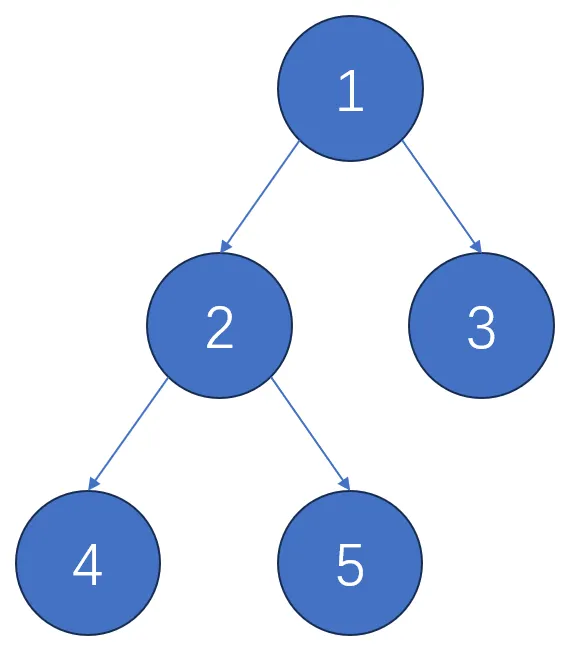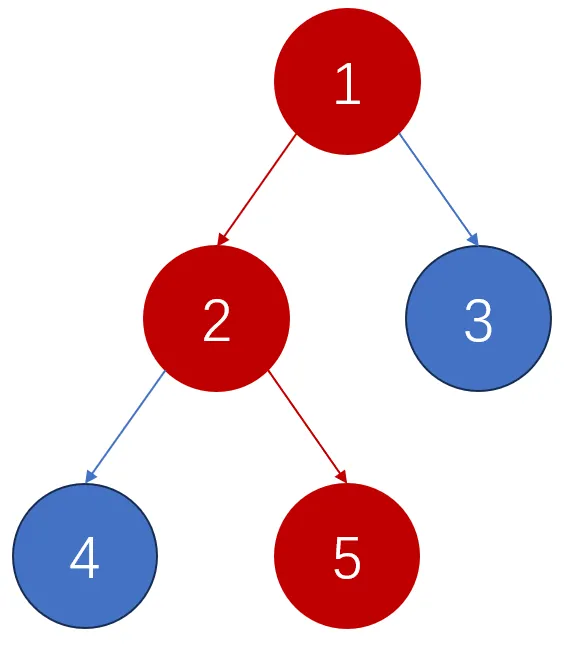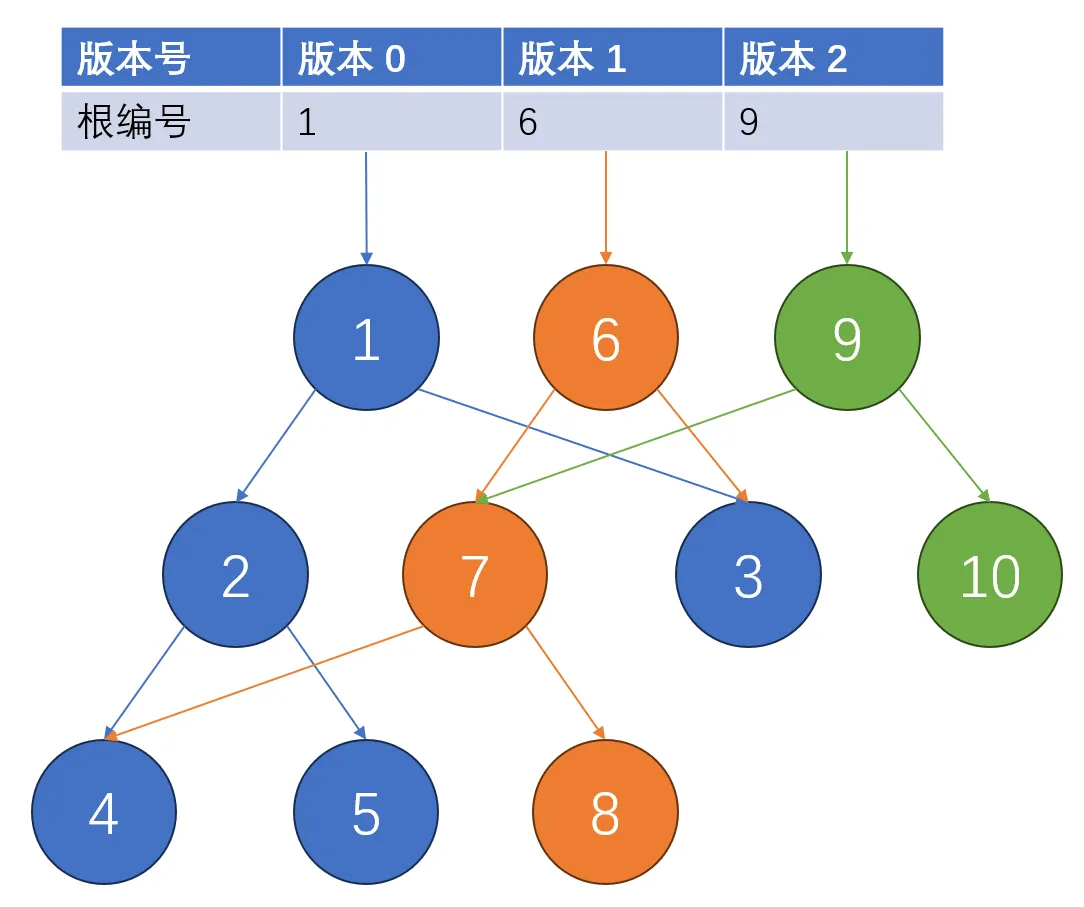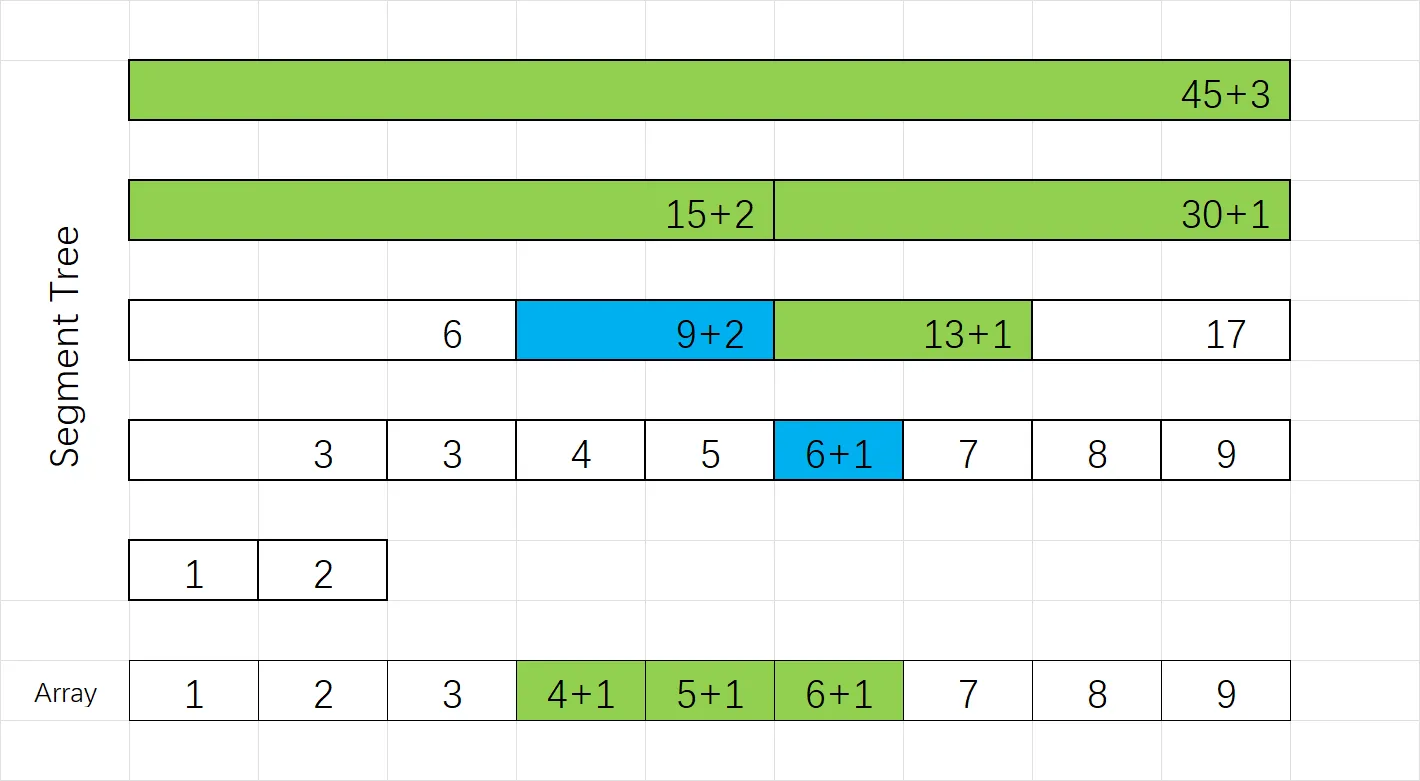# 1 可持久化线段树

## 1.1 问题引入

• 对于第 $x$ 个版本，修改 $a_l,a_{l+1},\dots,a_r$ 的值，作为一个新版本。
• 对于第 $x$ 个版本，查询 $a_l,a_{l+1},\dots,a_r$ 的和。

## 1.1 原理• 版本 $0$ $\to$ 修改节点 $5$ 的值 $\to$ 版本 $1$
• 版本 $1$ $\to$ 修改节点 $3$ 的值 $\to$ 版本 $2$## 1.2 实现

namespace pst
{
/* ### array index must start from ONE ### */
constexpr int MAXN = 1e6;
int n;
int root[MAXN];
int val[(MAXN << 5) + 10], lson[(MAXN << 5) + 10], rson[(MAXN << 5) + 10], cur_idx = 0;

int build(const vector<int> &arr, int s, int t)
{
int now = ++cur_idx;
if (s == t)
{
val[now] = arr[s];
return now;
}
int m = (s + t) / 2;
lson[now] = build(arr, s, m);
rson[now] = build(arr, m + 1, t);
return now;
}

int clone_node(int orig)
{
++cur_idx;
val[cur_idx] = val[orig];
lson[cur_idx] = lson[orig];
rson[cur_idx] = rson[orig];
return cur_idx;
}

int update(int x, int c, int s, int t, int p)
{
int now = clone_node(p);
if (s == t)
{
val[now] = c;
return now;
}
int m = (s + t) / 2;
if (x <= m)
lson[now] = update(x, c, s, m, lson[now]);
else
rson[now] = update(x, c, m + 1, t, rson[now]);
return now;
}

int query(int x, int s, int t, int p)
{
if (s == t)
return val[p];
int m = (s + t) / 2;
if (x <= m)
return query(x, s, m, lson[p]);
else
return query(x, m + 1, t, rson[p]);
}
};

## 1.3 区间修改与标记永久化namespace pst
{
/* ### array index must start from ONE ### */
constexpr int MAXN = 1e6;
int n;
signed root[MAXN];
int cur_idx = 0;
int val[(MAXN << 5) + 10], mark[(MAXN << 5) + 10];
signed lson[(MAXN << 5) + 10], rson[(MAXN << 5) + 10];

int build(const vector<int> &arr, int s, int t)
{
int now = ++cur_idx;
if (s == t)
{
val[now] = arr[s];
return now;
}
int m = (s + t) / 2;
lson[now] = build(arr, s, m);
rson[now] = build(arr, m + 1, t);
val[now] = val[lson[now]] + val[rson[now]];
return now;
}

int clone_node(int orig)
{
++cur_idx;
val[cur_idx] = val[orig];
mark[cur_idx] = mark[orig];
lson[cur_idx] = lson[orig];
rson[cur_idx] = rson[orig];
return cur_idx;
}

int update(int l, int r, int c, int s, int t, int p)
{
int now = clone_node(p);
val[now] += (min(r, t) - max(l, s) + 1) * c;
if (l <= s && t <= r)
{
mark[now] += c;
return now;
}
int m = (s + t) / 2;
if (l <= m)
lson[now] = update(l, r, c, s, m, lson[now]);
if (r > m)
rson[now] = update(l, r, c, m + 1, t, rson[now]);
return now;
}

int query(int l, int r, int s, int t, int p, int mk = 0)
{
if (l <= s && t <= r)
return val[p] + mk * (t - s + 1);
int m = (s + t) / 2, ans = 0;
if (l <= m)
ans += query(l, r, s, m, lson[p], mk + mark[p]);
if (r > m)
ans += query(l, r, m + 1, t, rson[p], mk + mark[p]);
return ans;
}
};

# 2 可持久化权值线段树（主席树）

## 2.1 普通线段树和权值线段树

• 普通线段树：维护信息，按个数开空间，维护具体信息。
• 权值线段树：维护桶，按值域（可离散化处理），维护个数。

## 2.3 原理

• 先找根节点的左儿子的 $r$ 版本的值，即代表 $r$ 版本时处于 $[low,mid]$ 的数的个数，记为 $sum_r$.
• 再找根节点的左儿子的 $l - 1$ 版本的值，即代表 $l-1$ 版本时处于 $[low,mid]$ 的数的个数，记为 $sum_{l-1}$.

• 如果 $x\geq k$，说明 $[low,mid]$ 范围包含的范围比我们要找的范围要大，因此我们递归进入左子节点更细致地查找。
• 如果 $x<k$，说明 $[low,mid]$ 范围包含了 $[l,r]$ 内前 $x$ 小的数，我们要找的是前 $k$ 小的数，那么我们应该再递归进入右子节点找前 $k-x$ 小的数，就相当于原区间的第 $k$ 小的数。

## 2.4 实现

namespace hjt
{
/* ### array index must start from ONE ### */

constexpr int MAXN = 1e6;
int n;
int sum[(MAXN << 5) + 10], lson[(MAXN << 5) + 10], rson[(MAXN << 5) + 10], cur_idx = 0;
int root[MAXN], cur_ver = 0;

int build(int s, int t)
{
int now = ++cur_idx;
if (s == t)
{
sum[now] = 0;
return now;
}
int m = (s + t) / 2;
lson[now] = build(s, m);
rson[now] = build(m + 1, t);
return now;
}

int clone_node(int orig)
{
++cur_idx;
sum[cur_idx] = sum[orig] + 1;
lson[cur_idx] = lson[orig];
rson[cur_idx] = rson[orig];
return cur_idx;
}

int update(int x, int s, int t, int p)
{
int now = clone_node(p);
if (s == t)
return now;
int m = (s + t) / 2;
if (x <= m)
lson[now] = update(x, s, m, lson[now]);
else
rson[now] = update(x, m + 1, t, rson[now]);
return now;
}

int query(int x, int l, int r, int s, int t)
{
if (s == t)
return s;
int delt = sum[lson[r]] - sum[lson[l]];
int m = (s + t) / 2;
if (x <= delt)
return query(x, lson[l], lson[r], s, m);
else
return query(x - delt, rson[l], rson[r], m + 1, t);
}
};

solve() 函数也附上，防止不知道怎么用，对应的是模板题：https://www.luogu.com.cn/problem/P3834

void solve()
{
int n, m;
cin >> n >> m;
vector<int> a(n + 10);
for (int i = 1; i <= n; i++)
cin >> a[i];
auto b = a;
sort(b.begin() + 1, b.begin() + 1 + n);
int uni = unique(b.begin() + 1, b.begin() + 1 + n) - b.begin() - 1;
hjt::root = hjt::build(1, uni);
for (int i = 1; i <= n; i++)
{
int t = lower_bound(b.begin() + 1, b.begin() + 1 + uni, a[i]) - b.begin();
hjt::root[i] = hjt::update(t, 1, m, hjt::root[i - 1]);
}
for (int i = 1; i <= m; i++)
{
int l, r, k;
cin >> l >> r >> k;
int t = hjt::query(k, hjt::root[l - 1], hjt::root[r], 1, m);
cout << b[t] << endl;
}
}